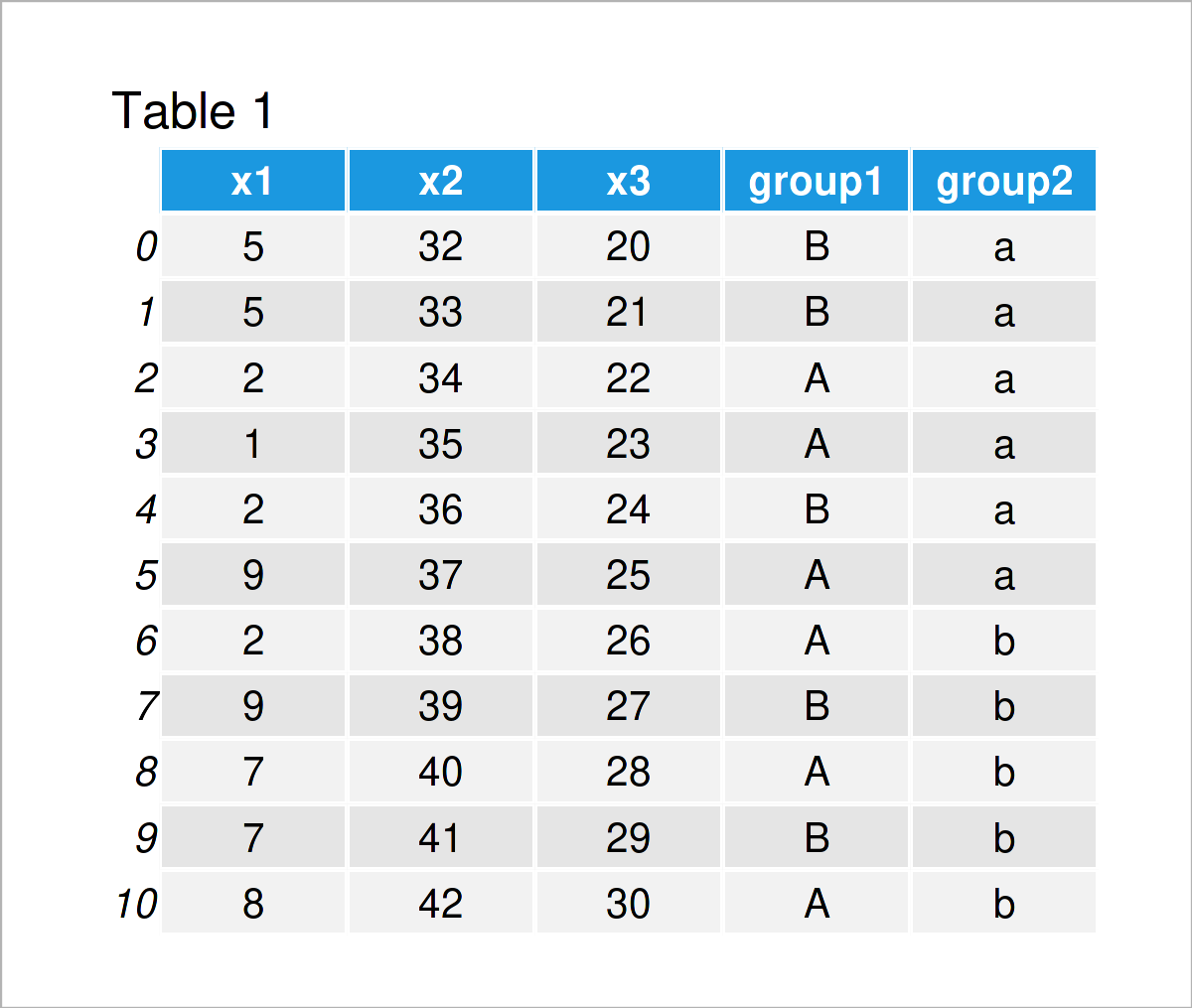# Variance by Group in Python (2 Examples)

In this Python post you’ll learn how to find the variance by group.

The tutorial contains these contents:

Let’s do this!

## Example Data & Add-On Libraries

To be able to use the functions of the pandas library, we first need to load pandas:

`import pandas as pd                                           # Import pandas library to Python`

We’ll use the following data as a basis for this Python programming language tutorial:

```data = pd.DataFrame({'x1':[5, 5, 2, 1, 2, 9, 2, 9, 7, 7, 8],  # Create pandas DataFrame
'x2':range(32, 43),
'x3':range(20, 31),
'group1':['B', 'B', 'A', 'A', 'B', 'A', 'A', 'B', 'A', 'B', 'A'],
'group2':['a', 'a', 'a', 'a', 'a', 'a', 'b', 'b', 'b', 'b', 'b']})
print(data)                                                   # Print pandas DataFrame```Have a look at the table that has been returned after executing the previous Python syntax. It shows that our pandas DataFrame has eleven rows and five columns.

The variables x1, x2, and x3 are floats and the variables group1 and group2 are our group and subgroup indicators.

## Example 1: Variance by Group in pandas DataFrame

In this example, I’ll explain how to calculate the variance by group.

For this task, we have to apply the groupby and var functions as shown below:

```print(data.groupby('group1').var())                           # Get variance by group
#                x1         x2         x3
# group1
# A       12.566667   9.066667   9.066667
# B        6.800000  14.700000  14.700000```

The output above shows the variance for each of our columns and each of our groups in the grouping variable group1.

Note that this output reflects population variances. In case you want to compute the sample variance instead, you would have to set the ddof argument of the std function to 1.

## Example 2: Variance by Group & Subgroup in pandas DataFrame

In Example 2, I’ll demonstrate how to compute the variance group-wise for each subgroup using two different grouping columns.

For this, we have to specify a list of all group column names within the groupby function:

```print(data.groupby(['group1', 'group2']).var())               # Get variance by multiple groups
#                       x1        x2        x3
# group1 group2
# A      a       19.000000  2.333333  2.333333
#        b       10.333333  4.000000  4.000000
# B      a        3.000000  4.333333  4.333333
#        b        2.000000  2.000000  2.000000```

The previous output shows separate variance results for each subgroup in our data set.

## Video & Further Resources

Do you need further information on the content of this tutorial? Then you may want to watch the following video which I have published on my YouTube channel. I’m explaining the Python codes of this page in the video:

Please accept YouTube cookies to play this video. By accepting you will be accessing content from YouTube, a service provided by an external third party.If you accept this notice, your choice will be saved and the page will refresh.

Furthermore, you could have a look at the related articles on my website.

At this point of the tutorial you should know how to calculate the variance by group in Python programming. In case you have further questions and/or comments, let me know in the comments section.

Subscribe to the Statistics Globe Newsletter